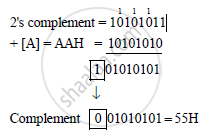Advertisement Remove all ads

# The Accumulator Contains Aa H and Register C Contains 55 H. What Will Be the Contents of Accumulator If Following Instructions Are Executed Independently? I) Cmp C Ii) Ana C Iii) Ora C Iv) Sub C - Computer Science 2

Answer in Brief

The accumulator contains AA H and register C contains 55 H. What will be the contents of accumulator if following instructions are executed independently?
i) CMP C
ii) ANA C
iii) ORA C
iv) SUB C

Advertisement Remove all ads

#### Solution

(i) CMP C
Compare with accumulator before executions :
[A] = AAH = 10101010
[C] = 55H = 01010101
Instructions : CMPC
After execution :
CY = 0, Z = 0
and [A] = AAH = 10101010

(ii) ANA C
Logical AND with accumulator
Before execution :
[A] = AAH = 10101010
[C] = 55H = 01010101
Instruction : ANA C
∴ AAH 10101010
AND 55H = 01010101
00000000 = OOH
After executions :
[A] = 00H

(iii) ORA C
Logically OR with accumulator
Before execution :
[A] = AAH = 10101010
[C] = 55H = 01010101
Instruction : ORA C
∴   AAH = 10101010
OR 55H = 01010101
11111111 = FFH

(iv) SUB C
Subtract C reg. from accumulator.
Before execution :
[A] = AAH = 10101010
[C] = 55H = 01010101
Instruction : SUB C
[C] = 55 H = 01010101
1’s complement 10101010
+         1carry
Result : [A] = 55H
Flags : S = 0, Z = 0, AC = 1, P = 1, CY = 0

Concept: Instruction Set and Programming of 8085
Is there an error in this question or solution?
Advertisement Remove all ads

#### APPEARS IN

Advertisement Remove all ads
Advertisement Remove all ads
Share
Notifications

View all notifications

Forgot password?